Updated by Leigh Ann Hull on Feb 05, 2018REPORTLeigh Ann Hull
Owner
8 items   2 followers   0 votes   77 views

# 5th Grade Math Resources: Decimal and Fractions

Here are some great resources for you to use when learning and practicing how to use decimals and fractions in operations.

1

## Multiplying decimals example (video) | Khan Academy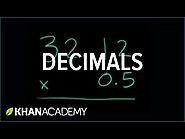This short video from Khan Academy gives a step by step audio and visual representation of how to multiply decimals. This is a good resource when you are in the beginning stages of learning to multiply decimals and can reinforce skills you have just been introduced to.

2

## Fractions to decimals song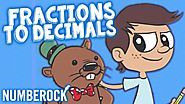This video sings you through converting fractions to decimals. Learn how to convert fractions, decimals, and percents with effective conversion strategies, and understand fractions as division with a song you are sure to remember. This video is great for students just beginning using fractions and decimals to perform operations.

3

## Practice Fifth grade math online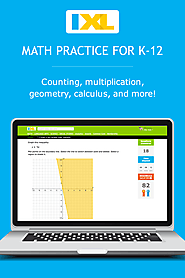IXL is a great resource for all math skills. This link will take you to the 5th grade page where you have access to hundreds of skill building activities. This website is designed for students at all skill levels and is great for reinforcement or moving ahead.

4

## Decimal Chart Patterns | MathPlayground.com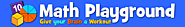Decimal chart patterns is an interactive game board that allows you to explore decimals using number patterns. This game is ideal for students who have a solid understanding of decimals. This game is exploratory, fun, and challenging.

5

## The "Decifractator"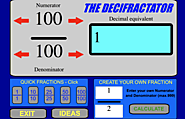The "DECIFRACTATOR" is an interactive calculator that allows you to convert fractions to decimals. This provides an opportunity for you to explore fraction and decimal equivalents.

6

## Dividing Decimals using Models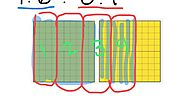This link provides a teaching video with picture and audio that shows how to divide decimals using models. The instructor takes you step by step through the solving the decimal division problem while using picture representation. This video is a good resource when you are in the early learning stage of how to divide decimals and before you are ready to work independently on decimal division problems.

7

## Division with Decimals - Grade 5 - Practice with Math Games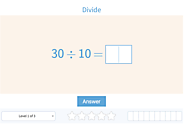This link takes you to an interactive website that gives division with decimal practice problems and immediate feedback. This activity is good practice when you feel confident to work independently with decimal division.

8

## Finding Fractions of Sets: StudyJams! Math | Scholastic.com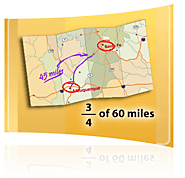This link takes you to an interactive Scholastic website where you will complete a word problem containing fractions with guided help. This activity walks you step-by-step through a real-world word problem using fractions. This is an activity that can help you understand how fractions work within word problem and how to approach the problem.